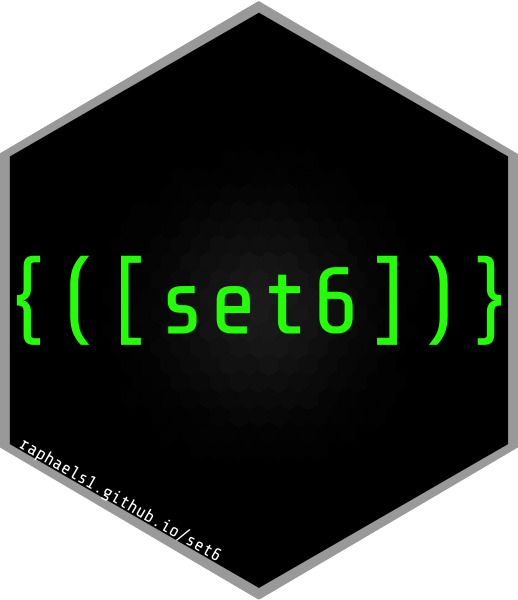## What is set6?

set6 is an R6 upgrade to the sets package in R that includes:

• Multi-dimensional sets
• Tuples
• Finite and infinite intervals
• Fuzzy sets and tuples
• Set operations including union, intersect, (asymmetric and symmetric) difference, and product
• Symbolic representation of infinite sets including common special sets such as the Reals and Integers
• ConditionalSets for defining sets according to logical conditions

## Installation

The current CRAN release can be installed with

install.packages("set6")

Or for the latest stable build

remotes::install_github("xoopR/set6")

## Main Features

### A Clear Inheritance Structure

# Sets require elements to be unique and order doesn't matter
Set$new(1, 2, 1) == Set$new(1, 2)
#>  TRUE
Set$new(1, 2) == Set$new(2, 1)
#>  TRUE

# But tuples can enforce these restrictions
Tuple$new(1, 2, 1) != Tuple$new(1, 2)
#>  TRUE
Tuple$new(1, 2) != Tuple$new(2, 1)
#>  TRUE

# Fuzzy sets and tuples extend sets further
f = FuzzySet$new(1, 0, 2, 0.6, 3, 1) f$inclusion(1)
#>  "Not Included"
f$inclusion(2) #>  "Partially Included" f$inclusion(3)
#>  "Fully Included"

# Symbolic intervals provide a clean way to represent infinite sets
Interval$new() #> [-∞,+∞] # Different closure types and classes are possible Interval$new(1, 7, type = "(]") # half-open
#> (1,7]
Interval$new(1, 7, class = "integer") == Set$new(1:7)
#>  TRUE

# And SpecialSets inheriting from these intervals
Reals$new() #> ℝ PosRationals$new()
#> ℚ+

### Set operations

# Union
Set$new(1, 4, "a", "b") + Set$new(5)
#> {1, 4,...,b, 5}
Interval$new(1, 5) + FuzzyTuple$new(1, 0.6)
#> [1,5]

# Power
Set$new(1:5)^2 #> {1, 2,...,4, 5}^2 # A symbolic representation is also possible setpower(Set$new(1:5), power = 2, simplify = FALSE)
#> {1, 2,...,4, 5}^2
Reals$new()^5 #> ℝ^5 # Product Set$new(1,2) * Set$new(5, 6) #> {1, 2} × {5, 6} Interval$new(1,5) * Tuple$new(3) #> [1,5] × (3) # Intersection Set$new(1:5) & Set$new(4:10) #> {4, 5} ConditionalSet$new(function(x) x == 0) & Set$new(-2:2) #> {0} Interval$new(1, 10) & Set$new(5:6) #> {5, 6} # Difference Interval$new(1, 10) - Set$new(5) #> [1,5) ∪ (5,10] Set$new(1:5) - Set$new(2:3) #> {1, 4, 5} ### Containedness and Comparators Interval$new(1, 10)$contains(5) #>  TRUE # check multiple elements Interval$new(1, 10)$contains(8:12) #>  TRUE TRUE TRUE FALSE FALSE # only return TRUE if all are TRUE Interval$new(1, 10)$contains(8:12, all = TRUE) #>  FALSE # decide whether open bounds should be included Interval$new(1, 10, type = "()")$contains(10, bound = TRUE) #>  TRUE Interval$new(1, 10, type = "()")$contains(10, bound = TRUE) #>  TRUE Interval$new(1, 5, class = "numeric")$equals(Set$new(1:5))
#>  FALSE
Interval$new(1, 5, class = "integer")$equals(Set$new(1:5)) #>  TRUE Set$new(1) == FuzzySet$new(1, 1) #>  TRUE # proper subsets Set$new(1:3)$isSubset(Set$new(1), proper = TRUE)
#>  TRUE
Set$new(1) < Set$new(1:3)
#>  TRUE

# (non-proper) subsets
Set$new(1:3)$isSubset(Set$new(1:3), proper = FALSE) #>  TRUE Set$new(1:3) <= Set$new(1:3) #>  TRUE # multi-dimensional checks x = PosReals$new()^2
x$contains(list(Tuple$new(1, 1), Tuple\$new(-2, 3)))
#>   TRUE FALSE

## Usage

The primary use-cases of set6 are:

1. Upgrading sets Extend the R sets package to R6, which allows for generalised Set objects with a clear inheritance structure. As well as adding features including symbolic representation of infinite sets, and cartesian products.
2. Defining parameter interfaces All objects inheriting from the Set parent class include methods equals and contains, which are used to check if two sets are equal or if elements lie in the given set. This makes set6 ideal for parameter interfaces in which a range of values (possibly multi-dimensional or of mixed types) need to be defined.

## Short-term development plans

Whilst the set6 API is stable, it is considered ‘maturing’, and therefore whilst there are no plans for major updates, these may still occur. There are a few features and refactoring we plan on implementing before we consider the package to be in its first complete version. These mainly include

• Finalising all methods and fields - some are missing or possibly inaccurate for some wrappers. For example the cardinality of ComplementSets is imprecise at the moment.
• We are considering adding a simplify method to wrappers to reduce classes inheriting from SetWrapper to simpler sets. This allows users to perform operations with simplify = FALSE and then to change their mind.
• There are some inefficient loops implemented that will need refactoring for more efficient code.
• Adding more tutorials to make the interface easier for beginners, especially people new to R6

At a later stage we may consider adding Venn diagrams for visualisation of sets and intervals, but this is very low priority.

## Similar Packages

• sets - The sets package uses S3 to define some symbolic representaton of mathematical sets, tuple, intervals, and fuzzy variants. However the symbolic representation is not consistent throughout the package, does not allow for clear inspection of set/interval elements, and there is no support for multi-dimensional sets.

• BaseSet - The BaseSet package focuses on storing and analysing sets in a ‘tidy’ way, with more options for data storage in long and wide formats. The primary usage is neat and efficient inspection of finite sets, there is no support for infinite sets or symbolic representation.

## Contributing

As set6 is in its early stages, contributions are very welcome. If you have any ideas for good features please open an issue or create a pull request. Otherwise bug reports are very appreciated if you stumble across any broken code, these can be posted to the issue tracker. For updates on set6 follow/star this repo.